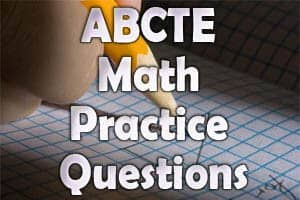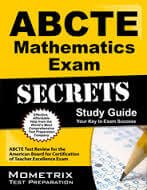# ABCTE Math Practice Questions

The ABCTE Math is an examination of the content typically found in introductory college math courses. This exam measures the readiness of applicant’s to become certified math teachers.  Time-wise, there are 240 minutes allowed. There are 125 multiple-choice questions which are divided into 7 content domains: (I) Number Sense, (II) Algebra and Functions, (III) Geometry and Measurement, (IV) Trigonometry, (V) Probability, Statistics, and Data Analysis, (VI) Linear Algebra, and (VII) Calculus.